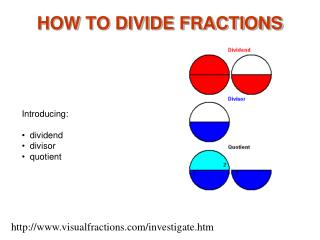DownloadDownload PresentationHOW TO DIVIDE FRACTIONS

# HOW TO DIVIDE FRACTIONS

Download Presentation## HOW TO DIVIDE FRACTIONS

- - - - - - - - - - - - - - - - - - - - - - - - - - - E N D - - - - - - - - - - - - - - - - - - - - - - - - - - -
##### Presentation Transcript

1. HOW TO DIVIDE FRACTIONS • Introducing: • dividend • divisor • quotient http://www.visualfractions.com/investigate.htm

2. Divide Fractions 1 Division is a form of subtraction. This picture shows that the divisor1/2 can be subtracted 3 times from the dividend 1 1/2 . A quotient 3 tells us how many times the divisor can be subtracted from the dividend.

3. Divide Fractions 2 To calculate the quotient, first write the dividend and divisor in fraction form. Then multiply 3/2 by the inverse of 1/2 . This gives a quotient of 3/2 x 2/1 or 3.

4. Divide Fractions 3 This picture shows that 1 3/4 can be subtracted from 5 1/4 three times.

5. Divide Fractions 4 The same example with number lines shows that 1 3/4 fits into 5 1/4 three times.

6. Divide Fractions 5 The divisorhas been decreased to 1 1/4. Notice the quotient is increased to 41/5. As the divisor decreases, the quotient increases.

7. Divide Fractions 6 The divisor has been decreased to 1. Notice the quotient is increased to 51/4. Dividing by 1 gives a quotient equal to the dividend.

8. Divide Fractions 7 When the divisor is less than 1, the quotient is larger than the dividend.

9. Divide Fractions 8 Decreasing the divisor to 1/2 increases the quotient to 10 1/2.

10. Divide Fractions 9 When the divisor is smaller than the dividend, the quotient is more than 1.

11. Divide Fractions 10 Another example where the divisor smaller than the dividend.

12. Divide Fractions 11 When the divisor is the same size as the dividend, the quotient is 1.

13. Divide Fractions 12 When the divisor is larger than the dividend, the quotient is less than 1.

14. Divide Fractions 13 Another example where the divisor is larger than the dividend.